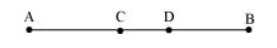# In the above question, point C is called a mid-point of line segment AB, prove that every line segment has one and only one mid-point.

Solution:

Let there be two mid-points, $C$ and $D$.$C$ is the mid-point of $A B$.

$\mathrm{AC}=\mathrm{CB}$

$\mathrm{AC}+\mathrm{AC}=\mathrm{BC}+\mathrm{AC}$ (Equals are added on both sides) $\ldots$ (1)

Here, $(B C+A C)$ coincides with $A B$. It is known that things which coincide with one another are equal to one another.

$\therefore \mathrm{BC}+\mathrm{AC}=\mathrm{AB} \ldots$(2)

It is also known that things which are equal to the same thing are equal to one another.

Therefore, from equations ( 1 ) and (2), we obtain

$\mathrm{AC}+\mathrm{AC}=\mathrm{AB}$

$\Rightarrow 2 \mathrm{AC}=\mathrm{AB} \ldots$(3)

Similarly, by taking $D$ as the mid-point of $A B$, it can be proved that

$2 \mathrm{AD}=\mathrm{AB} \ldots$(4)

From equation (3) and (4), we obtain

$2 A C=2 A D$ (Things which are equal to the same thing are equal to one another.)

$\Rightarrow A C=A D$ (Things which are double of the same things are equal to one another.)

This is possible only when point C and D are representing a single point.

Hence, our assumption is wrong and there can be only one mid-point of a given line segment.